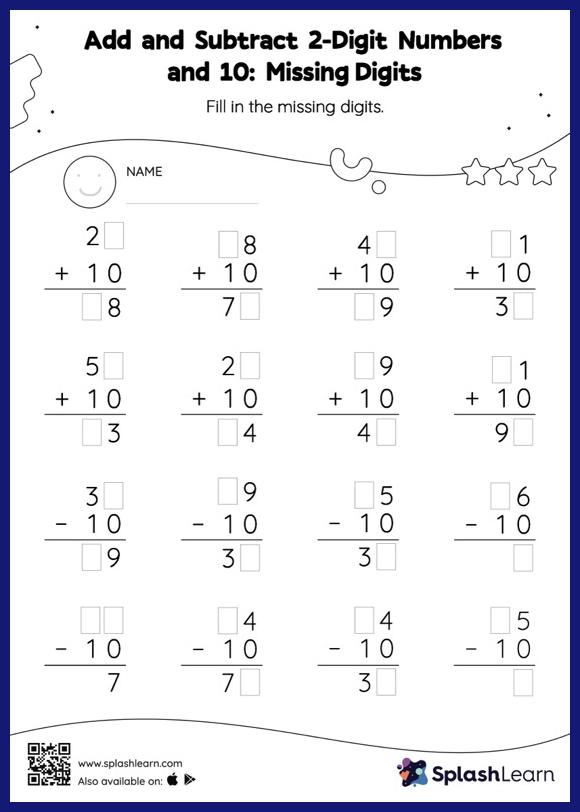# Add and Subtract 2-Digit Numbers and 10: Missing Digits Worksheet

Home > Add and Subtract 2-Digit Numbers and 10: Missing DigitsThis worksheet gives students an opportunity to apply the concepts of addition and subtraction to add and subtract 2-digit numbers and 10. Students use the count on or count back strategy or the relationship between addition and subtraction to find the missing number in this add and subtract 2-digit numbers and 10 worksheet.